# Math in Focus Grade 2 Chapter 7 Answer Key Metric Measurement of Length

Practice the problems of Math in Focus Grade 2 Workbook Answer Key Chapter 7 Metric Measurement of Length to score better marks in the exam.

## Math in Focus Grade 2 Chapter 7 Answer Key Metric Measurement of Length

Challenging Practice

Solve.

Question 1.
There are three drawings—A, B, and C. Drawing A is shown below.
________ Drawing A
Drawing B is 2 centimeters longer than Drawing A. Drawing C is 3 centimeters shorter than Drawing B. How long is Drawing C?
Drawing A is 2.5 cm,
Drawing B is 2 centimeters longer than Drawing A,
By adding 2 centimeters to 2.5 we get 4.5 cm as Drawing B,
Drawing C is 3 centimeters shorter than Drawing B,
By subtracting 3 cm from 4.5 cm we get 1.5 cm as Drawing C,
Therefore,Drawing A is 2.5cm,Drawing B is 4.5cm and Drawing C is 1.5cm.

Question 2.
Sara bought three pieces of ribbon. She bought 90 centimeters of ribbon in all. Check (✓) to show which three pieces of ribbon she bought.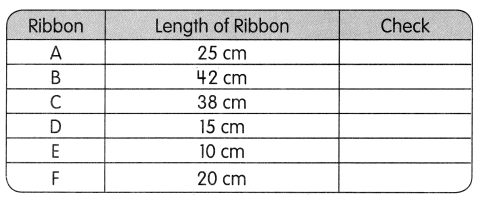Given,
Sara bought three pieces of ribbon,
She bought 90 centimeters of ribbon,
By adding 42 with 38 we get 80 and adding 80 with 10 we get 90,
Therefore, first ribbon is 42 cm, second ribbon is 38 cm and third ribbon is 10 cm.

Problem Solving

The picture shows the shadows of two trees.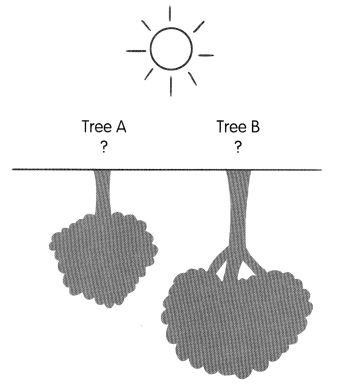Look at the shadows. Which tree is taller? Tree ____
___________________
___________________
___________________
Answer: Tree B is taller than Tree A
Explanation:
Given,
Tree A, Tree B Shadows and a Sun,
Tree B’s shadow is bigger than Tree A,
Therefore, Tree B is taller than Tree A.

Chapter Review/Test

Vocabulary

Fill in the blanks with the words in the box.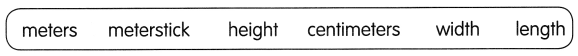Question 1.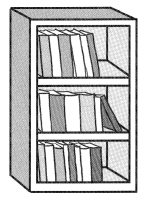To find how high this bookcase is, I need to find its ___________________
Answer: To find how high this bookcase is, I need to find its Height.

Question 2.
An earthworm is about 10 ____ long.

Question 3.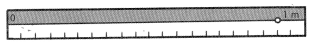This is a ________

Fill in the blanks with the words in the box.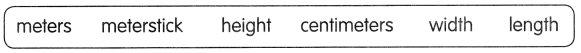Question 4.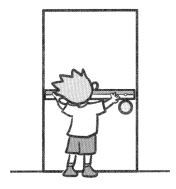Jose is trying to find the ___ of the door.
Answer: Jose is trying to find the width of the door.

Question 5.
A whiteboard is about 3 ____ long

Question 6.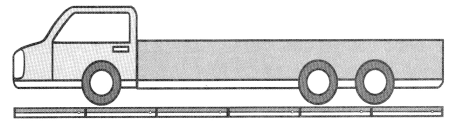The _________ of this truck is about 6 meters.

Concepts and Skills

Question 7.
What is the length of your math textbook?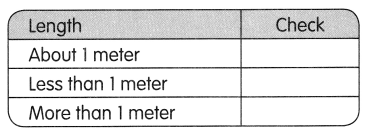Question 8.
What is the height of your desk?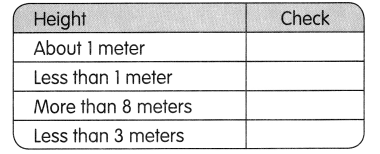Question 9.
What is the height of your classroom?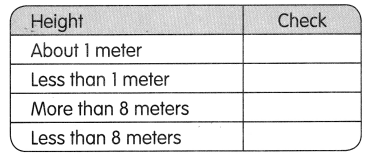Look at the objects measured. Then fill in the blanks.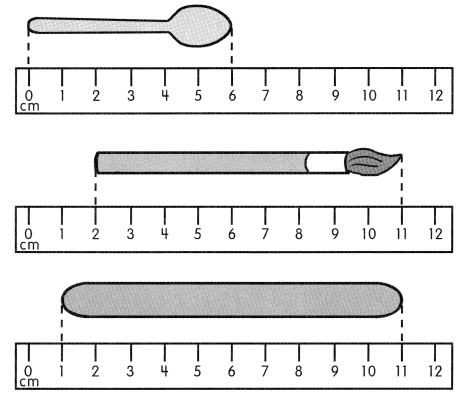Question 10.
spoon: ___ cm

Question 11.
brush: ___ cm

Question 12.
craftstick: ___ cm

Question 13.
The craftstick is ___ centimeters longer than the spoon.
The craftstick is 4 centimeters longer than spoon.
Explanation:
Given,
Craftstick is 10 centimeters,
Spoon is 6 centimeters,
By subtracting 6 from 10 we get 4,
Therefore, the craftstick is 4 centimeters longer than spoon.

Question 14.
The ___ is the shortest.
Answer: The Spoon is the shortest.
Explanation:
Given,
Spoon is 6 centimeters,
Brush is 9 centimeters,
Craftstick is 10 centimeters.

Solve.

Question 15.
Shane has 5 meters of cloth. He needs 16 meters more cloth to make some curtains. How many meters of cloth are needed to make the curtains?
____ meters of cloth are needed to make the curtains.
21 meters of cloth are needed to make the curtains.
Explanation:
Given,
Shane has 5 meters of cloth,
He needs 16 meters more cloth,
By adding 5 with 16 we get 21,
Therefore, 21 meters of cloth are needed to make the curtains.

Question 16.
Two boards are 26 meters long altogether. One board is 8 meters long. How long is the other board?
The other board is ____ meters long.
The other board is 18 meters long.
Explanation:
Given,
Two boards are 26 meters long altogether,
One board is 8 meters long,
By subtracting 8 from 26 we get 18,
Therefore, the other board is 18 meters long.

Question 17.
Bella is 161 centimeters tall. She is 12 centimeters taller than Joshua. How tall is Joshua?
Joshua is ___ centimeters tall.
Joshua is 149 centimeters tall.
Explanation:
Given,
Bella is 161 centimeters tall,
She is 12 centimeters taller than Joshua,
By subtracting 12 from 161 we get 149,
Therefore, Joshua is 149 centimeters tall.

Question 18.
Raul has a box that is 9 centimeters wide. Ling’s box is 3 centimeters wider than Raul’s box. Will both their boxes fit on a shelf that is 30 centimeters wide? Explain why.